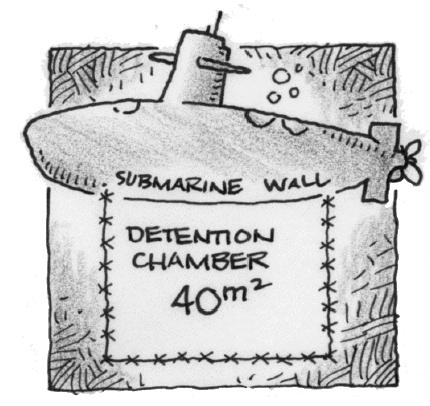### Home > CALC > Chapter 5 > Lesson 5.1.4 > Problem5-39

5-39.

YELLOW SUBMARINE, Part One

Ring O. Star was sick and tired of chasing blue meanies around the inside of his yellow submarine, so he decided to build a special detention chamber to hold the ones he caught. The first design he thought of was a rectangular chamber with sides made of razor-sharp barbed wire. To save money, he decided to build the chamber up against a submarine wall - that way only three sides needed to be made out of barbed wire. He estimated the detention chamber should have $40$ square meters of area. What dimensions should the chamber have so that Mr. Star can buy the least amount of barbed wire?You are being asked to 'minimize' perimeter. Did you recognize that this is an optimization problem?
The first thing you will do is build a geometric equation that represents the situation. Then you will apply Calculus.

What do we know?

Now that we have an equation, $P\left(h\right)$, let's find it's minimum value.
$P^\prime(h)$ = ___________________
To find the $h$-value of the max and min, set $P^\prime(h) = 0$, and solve for $h$.
Then, for a complete answer, don't forget to find the corresponding value of $b$.